1. /
2. CBSE
3. /
4. Class 11
5. /
6. Chemistry
7. /
8. CBSE Sample Papers Class...

# CBSE Sample Papers Class 11 Chemistry 2023### myCBSEguide App

Download the app to get CBSE Sample Papers 2023-24, NCERT Solutions (Revised), Most Important Questions, Previous Year Question Bank, Mock Tests, and Detailed Notes.

## CBSE Sample Question Paper for Class 11 Chemistry – in PDF

The new marking scheme and blueprint for Class 11 have been released by CBSE. We are providing Chemistry sample papers for Class 11 CBSE exams. These Sample Papers are available for free download in the myCBSEguide app and website in PDF format. CBSE Sample Papers for class 11 Chemistry with solutions will help students to score high in exams. We are also providing CBSE marking scheme and blueprint along with the Sample Papers. This helps students find answers to the most frequently asked questions and they will understand how to prepare for the CBSE exams. CBSE Sample Papers of Class 11 Chemistry for session 2022-23 are also available on the myCBSEguide app. Download the app today to get the latest and up-to-date study material.

## CBSE Sample Papers Class 11 Chemistry

We at myCBSEguide provide CBSE class 11 Sample Papers of chemistry for the years 2022, 2023 and 2024 with solutions in PDF format for free download. Students must download and practice the CBSE Sample Papers, and questions from NCERT books which are based on the latest syllabus issued by CBSE. Class 11 Chemistry New Sample Papers follow the blueprint of the current academic session only. All the students must check the latest syllabus and marking scheme. Sample papers for Class 11 Chemistry and other subjects are available for download as PDFs in the myCBSEguide app too. myCBSEguide provides sample papers with solutions for the session 2022-23.

## Class 11 – Chemistry Question Paper

Sample Paper – 01 (2022-23)

Maximum Marks: 70
Time Allowed: : 3 hours

General Instructions:

1. There are 35 questions in this question paper with internal choice.
2. SECTION A consists of 18 multiple-choice questions carrying 1 mark each.
3. SECTION B consists of 7 very short answer questions carrying 2 marks each.
4. SECTION C consists of 5 short answer questions carrying 3 marks each.
5. SECTION D consists of 2 case- based questions carrying 4 marks each.
6. SECTION E consists of 3 long answer questions carrying 5 marks each.
7. All questions are compulsory.
8. Use of log tables and calculators is not allowed.

1. ### Class 11 Chemistry Sample Paper Section A

2. There are ___ in 2000 mm?
a) 2 m
b) 0.002 m
c) 0.02 m
d) 200 m
3. Photoelectric effect established that light
a) behaves like particles
b) behaves like magnetic fields
c) behaves like waves
d) behaves like rays
4. The atomic number of the element unnilennium is:
a) 102
b) 109
c) 119
d) 108
5. Which of the following is incorrect?
a) The O—O bond length in H2O2 is larger than that in O2F2.
b) None of these
c) The O—O bond length in H2O2 is very slightly smaller than in {tex}\mathrm{O}_{2}^{2-}{/tex} ion.
d) {tex}\mathrm{O}_{2}^{-}{/tex} and {tex}\mathrm{O}_{2}^{+}{/tex} species are paramagnetic and have the same number of unpaired electrons.
6. A reaction occurs spontaneously if:
1. T{tex}\Delta{/tex}S = {tex}\Delta{/tex}H and both {tex}\Delta{/tex}H and {tex}\Delta{/tex}S are positive
2. T{tex}\Delta{/tex}S > {tex}\Delta{/tex}H and both {tex}\Delta{/tex}H and {tex}\Delta{/tex}S are positive
3. T{tex}\Delta{/tex}S < {tex}\Delta{/tex}H and both {tex}\Delta{/tex}H and {tex}\Delta{/tex}S are positive
4. T{tex}\Delta{/tex}S > {tex}\Delta{/tex}H and {tex}\Delta{/tex}H is positive and {tex}\Delta{/tex}S is negative
a) i and ii
b) iv and i
c) iii and iv
d) only ii

To practice more questions & prepare well for exams, download myCBSEguide App. It provides complete study material for CBSE, NCERT, JEE (main), NEET-UG and NDA exams. Teachers can use Examin8 App to create similar papers with their own name and logo.

7. Which of the following information can be obtained on the basis of Le Chatelier’s principle?
a) Shift in equilibrium position on changing value of a variable
b) Equilibrium constant of a chemical reaction
c) Entropy change in a reaction
d) Dissociation constant of a weak acid
8. If an atom is reduced, its oxidation number
a) First increases then decreases
b) Decreases
c) Increases
d) Does not change
9. CH3 – MgBr and (C2H5)CuLi are ________.
a) electrophiles
b) lewis acids
c) nucleophiles
d) both electrophiles and nucleophiles
10. Which of the following is not an aromatic compound?
a)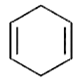b)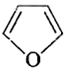c)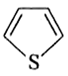d)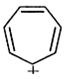11. The first element of a group in many ways differs from the other heavier members of the group. This is due to:
a) all of the these
b) the small size
c) the high electronegativity and high ionisation potential
d) the unavailability of d-orbitals
12. For vaporization of water at 1 atmospheric pressure, the values of {tex}\Delta{/tex}H and {tex}\Delta{/tex}S are 40.63 kJ mol-1 and 108.8JK-1 mol-1, respectively. The temperature when Gibbs energy change ({tex}\Delta{/tex}G) for this transformation will be zero, is:
a) 393.4 K
b) 373.4 K
c) 293.4 K
d) 273.4 K
13. Chloroprene is used in making:
a) All of these
b) plastic
c) petrol
d) synthetic rubber
14. Paper chromatography is an example of ________.
a) column chromatography
c) partition chromatography
d) thin-layer chromatography
15. Stereoisomeric alkenes due to the different arrangement of atoms or groups in space are referred to as:
a) Constitutional isomers
b) Position isomers
c) Chain isomers
d) Geometric isomers
16. Assertion (A): VIBGYOR signifies the seven colour of visible light.
Reason (R): Red colour corresponds to higher frequency and blue colour to lower frequency region.

a) Both A and R are true and R is the correct explanation of A.
b) Both A and R are true but R is not the correct explanation of A.
c) A is true but R is false.
d) A is false but R is true.
17. Assertion (A): Atoms can neither be created nor be destroyed.
Reason (R): Under similar conditions of temperature and pressure, an equal volume of gases does not contain an equal number of atoms.

a) Both A and R are true and R is the correct explanation of A.
b) Both A and R are true but R is not the correct explanation of A.
c) A is true but R is false.
d) A is false but R is true.
18. Assertion (A): The number of moles of KMnO4 reduced by one mole of KI in an alkaline medium is three.
Reason (R): The tendency of an electrode to lose electrons is known as oxidation potential.

a) Both A and R are true and R is the correct explanation of A.
b) Both A and R are true but R is not the correct explanation of A.
c) A is true but R is false.
d) A is false but R is true.
19. Assertion (A): NO ion is isoelectronic with CN ion.
Reason (R): Isoelectronic ions have same number of electrons.

a) Both A and R are true and R is the correct explanation of A.
b) Both A and R are true but R is not the correct explanation of A.
c) A is true but R is false.
d) A is false but R is true.
20. ### Class 11 Chemistry Sample Paper Section B

21. How much potassium chlorate should be heated to produce 2.24L of oxygen at NTP?
22. What happens when
1. bromoethane is treated with zinc and hydrochloric acid?
2. hydrogen is passed into 2-bromopropane in the presence of palladium?

OR

Propanal and pentane-3-one are the ozonolysis products of an alkene. What is the structural formula of the alkene?

23. 2 {tex}\times{/tex}108 atoms of carbon are arranged side by side. Calculate the radius of carbon if the length of this arrangement is 2.4 cm.

OR

Using s, p and d notations, describe the orbitals with following quantum numbers :

1. n = 1, l = 0
2. n = 4, l = 3
3. n = 3, l = 1
4. n = 4, l = 2
24. Explain the non-linear shape of H2S and non-planar shape of PCl3 using valence shell electron pair repulsion theory.
25. A man takes a diet equivalent to 10000 kJ per day and does work, in expanding his energy in all forms equivalent to 12500 kJ per day. What is the change in internal energy per day? If the energy lost was stored as sucrose (1632 kJ per 100 g), how many days should it take to lose 2 kg of his weight?
26. Increase in enthalpy of the surroundings is equal to decrease in enthalpy of the system. Will the temperature of system and surroundings be the same when they are in thermal equilibrium?
27. What is meant by electrochemical series? Write the characteristics of electrochemical series.
28. ### Class 11 Chemistry Sample Paper Section C

1. The energy associated with first orbit in hydrogen atom is -2.17{tex}\times{/tex}10-18.
2. What is the energy associated with the fifth orbit?
3. Calculate the radius of Bohr’s fifth orbit for the hydrogen atom.
29. Write the electronic configurations of the elements with atomic numbers 9,11,21 and 36. Stating reasons, predict the following from these configurations:
1. Which of them has the lowest ionization enthalpy?
2. Which of them has the highest negative electron gain enthalpy?
3. Which of them has positive electron gain enthalpy?
30. If two elements can combine to form more than one compound, the masses of one element that combine with a fixed mass of the other element, are in the whole-number ratio.
1. Is this statement true?
2. If yes, according to which law?
3. Give one example related to this law.
31. Suggest a method to purify
1. a liquid which decomposes at its boiling point.
2. kerosene oil containing water.
3. camphor containing traces of common salt.
32. Justify the following statements.
1. Reaction with negative Gibb’s energy always has an equilibrium constant greater than 1.
2. Many thermodynamically feasible reactions do not occur under ordinary conditions.
3. At low temperatures, enthalpy change dominates the {tex}\Delta G{/tex} expression and at high temperatures, it is the entropy that dominates the value of {tex}\Delta G{/tex}.

OR

1. Why standard entropy of an elementary substance is not zero whereas standard enthalpy of formation is taken as zero?
2. Under what conditions will the reaction occur, if
1. both {tex}\Delta{/tex}H and {tex}\Delta{/tex}S are positive
2. both {tex}\Delta{/tex}H and {tex}\Delta{/tex}S are negative
33. ### Class 11 Chemistry Sample Paper Section D

Alkanes, like propane and isobutane become alkenes like propylene and isobutylene through a chemical process called dehydrogenation, the removal of hydrogen, and the reverse of hydrogenation. The petrochemical industry often uses this process to create aromatics and styrene. The process is highly endothermic and requires temperatures of 932 degrees F, 500 degrees C and above. Common dehydrogenation processes include aromatization, in which chemists aromatize cyclohexene in the presence of hydrogenation acceptors using the elements sulfur and selenium, and the dehydrogenation of amines to nitriles using a reagent like iodine pentafluoride. Dehydrogenation processes can also convert saturated fats to unsaturated fats in the manufacturing of margarine and other foods. The chemical reactions during dehydrogenation are possible at high temperatures because the release of hydrogen gas increases the collapse of the system.{tex}H – \mathop C\limits_{\mathop |\limits_H }^{\mathop |\limits^H } – \mathop C\limits_{\mathop |\limits_H }^{\mathop |\limits^H } – \mathop C\limits_{\mathop |\limits_H }^{\mathop |\limits^H } – H{/tex}

1. Arrange the three isomeric pentanes in order of increasing stability at room temperature.
2. Give a method of preparation of propane from an alkene.
3. Give a method of preparation of propane from  an alkyl halide.
4. OR

Write the structure of all the alkenes that can be hydrogenated to form 2-methyl butane.

To practice more questions & prepare well for exams, download myCBSEguide App. It provides complete study material for CBSE, NCERT, JEE (main), NEET-UG and NDA exams. Teachers can use Examin8 App to create similar papers with their own name and logo.

In order to explain the characteristic geometrical shapes of polyatomic molecules, Pauling introduced the concept of hybridisation. The orbitals undergoing hybridisation should have nearly the same energy. There are various type of hybridisations involving s, p and d-type of orbitals. The type of hybridisation gives the characteristic shape of the molecule or ion.

1. Why all the orbitals in a set of hybridised orbitals have the same shape and energy?
2. Out of XeF2 and SF2 which molecule has the same shape as {tex}N O_{2}^{+}{/tex} ion?
3. Out of XeF4 and XeF2 which molecule doesn’t have the same type of hybridisation as P(Phosphorus) has in PF5?
4. OR

Unsaturated compounds undergo additional reactions. Why?

36. ### Class 11 Chemistry Sample Paper Section E

37. Discuss the principle of estimation of halogens, sulphur and phosporus present in an organic compound.

OR

Give a brief description of the principles of the following techniques taking an example in each case:

1. Crystallisation
2. Distillation
3. Chromatography
38. Calculate the pH of a 0.10M ammonia solution. Calculate the pH after 50.0 mL of this solution is treated with 25.0 mL of 0.10M HCl. The dissociation constant of ammonia, Kb = 1.77 {tex}\times{/tex} 10–5

OR

Equilibrium constant Kc for the reaction, {tex}{N_2}(g) + 3{H_2}(g) \rightleftharpoons 2N{H_3}(g){/tex} at 500 K is 0.061.
At a particular time, the analysis shows that the composition of the reaction mixture is: 3.0 mol L-1 of N2,. 2.0 mol L-1 of Hand 0.50 mol L-1 of NH3. Is the reaction at equilibrium? If not, in which direction does the reaction tend to proceed to reach the equilibrium?

1. Neutrons can be found in all atomic nuclei except in one case. Which is this atomic nucleus and what does it consist of?
2. On the basis of the equation pH = – log [H+], the pH of 10-8 mol dm-3 solution of HCl should be 8. However, it is observed to be less than 7.0. Explain the reason.
3. Which colour will appear in the Lassaigne’s test if the compound contains both nitrogen and sulphur.
4. Which of the two trans-but-2-ene or trans-pent-2-ene is non-polar?
5. Which orbital is non – directional?

## Class 11 – Chemistry Sample Paper Solution

Solution

1. ### Chemistry Paper Solution Section A

2. (a) 2 m
Explanation: Since, 1 mm =  0.001 m
{tex}\therefore{/tex}  2000 mm =  0.001 {tex}\times{/tex} 2000 =  2 m
3. (a) behaves like particles
Explanation: The emission of free electrons from a metal surface when light is shone on it is called the photoemission or the photoelectric effect. This effect led to the conclusion that light is made up of packets or quantum of energy. Einstein already associated the light quantum with momentum. This strongly supported the particle nature of light and these particles were named photons. Thus, the wave-particle duality of light came into the picture. Einstein won the Nobel Prize for Physics not for his work on relativity, but for explaining the photoelectric effect.
4. (b) 109
Explanation: un = 1
nil = 0
enn = 9
So, atomic number = 109
5. (b) None of these
Explanation: None of these
6. (d) only ii
Explanation: For spontaneous reaction {tex}\Delta{/tex}G = -ve
{tex}\Delta{/tex}G = {tex}\Delta{/tex}H – T{tex}\Delta{/tex}S
{tex}\Delta{/tex}H = +ve, {tex}\Delta{/tex}S = +ve and T{tex}\Delta{/tex}S > {tex}\Delta{/tex}H
To practice more questions & prepare well for exams, download myCBSEguide App. It provides complete study material for CBSE, NCERT, JEE (main), NEET-UG and NDA exams. Teachers can use Examin8 App to create similar papers with their own name and logo.
7. (a) Shift in equilibrium position on changing value of a variable
Explanation: According to Le Chatelier’s principle, if an equilibrium is subjected to a change in concentration, pressure or temperature, etc. equilibrium shifts in such a way so as to undo the effect of a change imposed.
8. (b) Decreases
Explanation: Reduction happens when an element gains electrons; in this case, its oxidation number is lowered.
9. (c) nucleophiles
Explanation: CH3 – Mgl {tex}\longrightarrow \underset {\text{Nucleophile}}{\overset -CH_3} + \overset + Mgl{/tex}
(C2H5)2CuLi {tex}\xrightarrow {-R-X}\underset {\text{Nucleophile}}{(C_2H_5){\overset -Cu}}{/tex} + LiX + R – C2H5
10. (a)Explanation: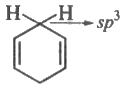compound contain non-planar atom, so it is non-aromatic.
11. (a) all of the these
Explanation: all of the these
12. (b) 373.4 K
Explanation: {tex}\Delta{/tex}G = {tex}\Delta{/tex}H – T{tex}\Delta{/tex}S
{tex}\Delta{/tex}G = 0, {tex}\therefore \ \Delta{/tex}H = T{tex}\Delta{/tex}S
T = {tex}\frac{\Delta H}{\Delta S}=\frac{40.63 \ \times \ 10^{3}}{108.8}{/tex} = 373.4 K
13. (d) synthetic rubber
Explanation: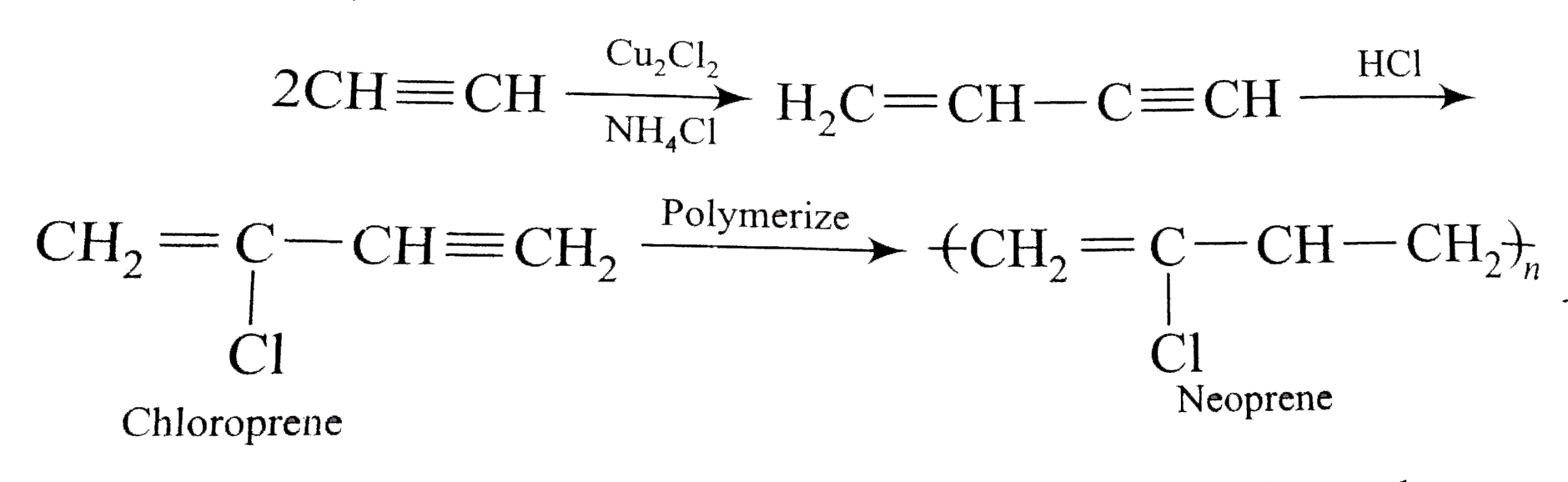Chloroprene readily polymerizes to a rubber-like substance known as neoprene.
14. (c) partition chromatography
Explanation: partition chromatography
15. (d) Geometric isomers
Explanation: Isomers which have the same structural formulae but differ in the relative spatial arrangements of atoms or groups around the double bond are called geometric isomers. Thus geometrical isomers are a type of stereoisomers.
16. (c) A is true but R is false.
Explanation: Visible light when passed through a prism splits into seven colours, Violet (V), Indigo (I), Blue (B), Green (G), Yellow (Y), Orange (O) and Red (R).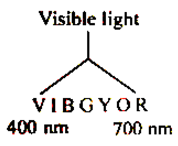{tex}\lambda=\frac{c}{v}{/tex}
Wavelength and frequency are inversely related. Red colour with higher wavelength will have lower frequency.
17. (c) A is true but R is false.
Explanation: Atoms can neither be created nor destroyed. This is the statement of the law of conservation of mass. Under similar conditions of temperature and pressure. An equal volume of gases contains an equal number of atoms.
As PV = nRT(Ideal gas equation) If, for I case, P1V1 = n1RT1
for II case, P2V2 = n2RT2
{tex}\Rightarrow \frac{P_{1} V_{1}}{P_{2} V_{2}}=\frac{n R T_{1}}{n_{2} R T_{2}} {/tex}
{tex}\Rightarrow{/tex} n= n2
18. (d) A is false but R is true.
Explanation: In an alkaline medium, the reduction of KMnO4 with KI will take place as
2KMnO4 + H2{tex}\to{/tex} 2 KOH + 2 MnO2
KI + 3[O] {tex}\to{/tex} KIO3
Hence the overall reaction is
KI + 2KMnO4 + H2O {tex}\to{/tex} KIO3 + 2 KOH + 2 MnO2
So, one mole of KI will reduce two moles of KMnO4.
19. (d) A is false but R is true.
Explanation: NO = 7 + 8 + 1 = 16e whereas CN = 6 + 7 + 1 = 14e, So both are not isoelectronic.
20. ### Chemistry Paper Solution Section B

21. When potassium chlorate is heated, then following reaction takes place:
2KClO3 {tex}\rightarrow{/tex} 2KCl + 3O2
Molecular mass of 1mol KClO3 = 39 + 35.5 + 3 {tex}\times{/tex}16= 122.5 g
Molecular mass of 2 mols of KClO3 = 2{tex}\times{/tex}122.5 g =245 g
Volume occupied by 3 mol of oxygen = 3{tex}\times{/tex}22.4L = 67.5 L
Therefore, 67.2L of oxygen is produced from 245g of KClO3
2.24L of oxygen is produced from = {tex}\frac { 245 } { 67.2 } \times 2.24{/tex}= 8.17g of KClO3
1. Bromoethane when treated with zinc and hydrochloric acid, it is reduced to the Ethane.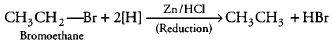2. 2-Bromopropane is treated with hydrogen in the presence of palladium, Pd, propane is obtained as a major product.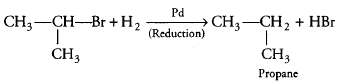OR

We can identify the structure of alkene, by writing the structure of propanal and Pentan-3-one side by side with their oxygen atom facing towards each other.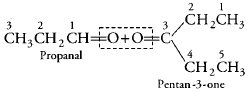Then remove the oxygen atom and join the two ends by double bond and thus, we obtain the structure of required alkene (3-ethylhex-3-ene).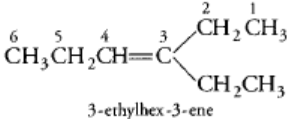22. The length of the arrangement = 2.4 cm
Total number of carbon atoms present = 2 {tex}\times{/tex}108
The diameter of each carbon atom = {tex}\frac{(2.4 \mathrm{cm})}{\left(2 \times 10^{8}\right)}=1.2 \times 10^{-8}{/tex} cm
The radius of each carbon atom ,= {tex}\frac{1}{2}\left(1.2 \times 10^{-8}\right){/tex}= 6.0 {tex}\times{/tex} 10-9 cm
= 0.06 nm

OR

1. n = 1, l = 0 : 1s orbital
2. n = 4, l = 3 : 4f orbital
3. n = 3, l = 1 : 3p orbital
4. n = 4, l = 2 : 4d orbital
23. In H2S, S belongs to group 16 has 6 valence electrons. It shares 1 valence electron with each H. Thus it has 2 bond electron pairs and 2 lone pairs of electrons. So it has bent shape instead of a linear shape due to lone pair-lone pair replusions and lone pair-bond pair repulsions.
In PCl3, P belongs to group 15 has 5 valence electrons. It shares 1 valence electron with each of the 3 Cl atoms. Thus, it has 3 bond pairs of electrons and 1 lone pair are present around the central atom. So it has trigonal pyramidal shape instead of trigonal planar shape due to lone pair-bond pair replusions.
24. According to the question, energy has taken by a man = 10000 kJ
Also, change in internal energy per day = 12500 – 10000 = 2500 kJ
Now, 100 g of sugar corresponds to energy = 1632 kJ loss in energy
2000 g of sugar corresponds to energy = {tex}\frac { 1632 \times 2000 } { 100 } = 32640 \ kJ{/tex}
Therefore, the number of days required to lose 2000 g of weight or 32640 kJ of energy = {tex}\frac { 32640 } { 2500 } {/tex} = 13 days.
25. Increase in enthalpy of the surroundings is equal to decrease in the enthalpy of the system.
If the system is in thermal equilibrium with the surroundings, then the temperature of the surroundings is same as that of the system.
26. The arrangement of various elements in the order of increasing values of standard reduction potentials is called  “Electrochemical series”..
Charecteristics of electrochemical series  :

• The substances which are stronger reducing agents than hydrogen are placed above hydrogen in the series and have negative values of standard electrode potentials.
• All those substances which have positive values of reduction potentials and placed below hydrogen in the series are weaker reducing agents.
• The substances which  are stronger oxidising agents  than the H+ ion are placed below hydrogen  in the series .
• Activity of metals decreases from top to bottom.
• The activity of nonmetals increases from top to bottom.
• Metals at the top have the tendency to loose electrons readily . These are active metals .
27. ### Chemistry Paper Solution Section C

1. For an electron, the energies in two orbits may be compared as:
{tex}\frac{E_{1}}{E_{2}}=\left(\frac{n_{2}}{n_{1}}\right)^{2}{/tex}
According to available data: n1 = 1,E1 = – 2.17 {tex}\times{/tex} 10-18 J atom-1, n2 = 5
2. {tex}\therefore{/tex} {tex}\frac{\left(-2.17 \times 10^{-18} \mathrm{J} \text { atom }^{-1}\right)}{\mathrm{E}_{2}}=\left(\frac{5}{1}\right)^{2}{/tex} = 255
E{tex}\frac{\left(-2.17 \times 10^{-18} \mathrm{J} \text { atom }^{-1}\right)}{25}{/tex}= -8.77 {tex}\times{/tex} 10-20 J
3. For hydrogen atom rn = 0.529 {tex}\times{/tex} n2 {tex}\stackrel{\circ}{A}{/tex}
r5 = 0.529 {tex}\times{/tex} (5)2 = 13.225 {tex}\stackrel{\circ}{A}{/tex} = 1.3225 nm
28. The electronic configurations of the elements with atomic numbers 9,11,21 and 36 are as follow:
Atomic number 9 (F): [He] 2s2 2p5
Atomic number 11 (Na): [Ne] 3s1
Atomic number 21 (Sc): [Ar] 3d1 4s2
Atomic number 36 (Kr): [Ar] 3d10 4s2 4p6

1. 11 atomic number has the lowest ionization enthalpy.
2. 9  atomic number has the highest negative electron gain enthalpy.
3. 36 atomic number has positive electron gain enthalpy
1. Yes, the statement is true.
2. According to the law of multiple proportions.
3. {tex}\mathop {{{\text{H}}_{\text{2}}}\,}\limits_{2\,\,{\text{g}}} {\text{ + }}\,\mathop {{{\text{O}}_{\text{2}}}}\limits_{16\,g} \, \to \,\mathop {{{\text{H}}_2}{\text{O}}}\limits_{18\,{\text{g}}} {/tex}
{tex}\mathop {{{\text{H}}_{\text{2}}}\,}\limits_{2\,\,{\text{g}}} {\text{ + }}\,\mathop {{{\text{O}}_{\text{2}}}}\limits_{32\,g} \, \to \,\mathop {{{\text{H}}_2}{\text{O}}}\limits_{34\,{\text{g}}} {/tex}
Here, masses of oxygen, (i.e., 16 g in H2O and 32 g in H2O2) which combine with a fixed mass of hydrogen (2g) are in the simple ratio i.e., 16: 32 or 1: 2.
1. Distillation under reduced pressure.
2. Since the two liquids are immiscible, the technique of solvent extraction with a separating funnel is used.
Kerosene being lighter than water forms the upper layer while water forms the lower layer.
The lower water layer is run off when kerosene oil is obtained. It is dried over anhydrous CaCl2 or MgSO4 and then distilled to give pure kerosene oil.
3. Sublimation Camphor sublimes while common salt remains as residue in the China dish.
1. We know that, {tex}\Delta G{/tex} = – 2.303 RT log K.
Thus, when {tex}\Delta G ^ { \circ }{/tex} < 0, K > 1.
2. Under ordinary conditions, the average energy of the reactants may be less than threshold energy. They requires some activation energy to initiate the reaction.
So, many thermodynamically feasible reactions do not occur under ordinary conditions.
3. We know that, {tex}\Delta G = \Delta H – T \Delta S{/tex}.
At low temperature, {tex}T \Delta S{/tex} is small.
Hence, {tex}\Delta H{/tex} dominates.
At high temperature, {tex}T \Delta S{/tex} is large.
Hence, {tex}\Delta S{/tex} dominates the value of {tex}\Delta G{/tex}.

OR

1. A substance has perfectly ordered arrangement of its constituent particles only at absolute zero. When the element from itself. This means no heat change.
Thus {tex}\Delta{/tex}fH = 0
1. If both {tex}\Delta{/tex}H and {tex}\Delta{/tex}S are positive {tex}\Delta{/tex}G can be -ve only in magnitude. Thus the temperature should be high.
2. If both {tex}\Delta{/tex}H and {tex}\Delta{/tex}S are negative {tex}\Delta{/tex}G can be negative only T{tex}\Delta{/tex}S < {tex}\Delta{/tex}H is magnitude. Thus the value of T should be low.
29. ### Chemistry Paper Solution Section D

1. The stability of structural isomers is directly proportional to branching. As the branching increases, the structure becomes more compact and this decreases molecular surface area per atom and so leads to a lowering of energy and increases in stability. Hence, correct order of stablity is:
Pentane, CH3(CH2)3CH3 < iso-pentane, (CH3)2CH CH2CH3 < neo-pentane, (CH3)4C
2. From alkene, alkane can be prepared by hydrogenation.
{tex}C{H_3} – CH = C{H_2} + {H_2}\xrightarrow[{catalyst}]{{Pt,Pd\,\,or\,\,Ni}}{/tex} {tex}C{H_3} – CH – C{H_3}{/tex}
3. From alkyl halides, alkane can be prepared by reduction in the presence of Zn/HCl or LiAlH4.
{tex}C{H_3} – C{H_2} – C{H_2} – Cl\xrightarrow[{or\,\,LiAl{H_4}}]{{Zn/HCl}}{/tex} {tex}C{H_3} – C{H_2} – C{H_3}{/tex}
4. OR

The alkenes must have the same carbon skeleton as 2-methyl butane.
{tex}C – \mathop C\limits_{\mathop |\limits_C } – C – C{/tex}
There are three different positions for the double bond; hence the three different alkenes are
{tex}C{H_2} = \mathop C\limits_{\mathop |\limits_{C{H_3}} } – C{H_2} – C{H_3}{/tex}{tex}C{H_3} – \mathop C\limits_{\mathop |\limits_{C{H_3}} } = CHC{H_3}{/tex}{tex}C{H_3} – \mathop {CH}\limits_{\mathop |\limits_{C{H_3}} } – CH = C{H_2}{/tex}

1. Hybrid orbitals are formed after combining atomic orbitals and have the equivalent shape and energy in the given set of hybridised orbitals.
2. XeF2 molecule has the same shape as {tex}N O_{2}^{+}{/tex} ion.
3. XeF4 molecule doesn’t have the same type of hybridisation as P(Phosphorus) has in PF5.
4. OR

Unsaturated hydrocarbon molecules include two- or three-fold bonds of carbon. The  {tex}\pi{/tex} -bond is a multiple bond, which becomes unstable and hence adds across numerous bonds.

30. ### Chemistry Paper Solution Section E

31. Estimation of halogens: It involves oxidising the organic substance with fuming nitric acid in the Presence of silver nitrate. The halogen of the substance is thus converted to silver halide which is separated and weighed:
Weight of organic compound = W gm
Weight of silver halide = x g.
% of halogen = {tex}\frac{\text { At. wt. of halogen } \times 100 \mathrm{x}}{\text { Mol. wt. of silver halide } \times \mathrm{w}}{/tex}
Estimation of sulphur: The organic substance is heated with fuming nitric acid but no silver nitrate is added. The sulphur of the substance is oxidised to sulphuric acid which is then precipitated as barium sulphate by adding excess of barium chloride solution. From the weight of BaSOso obtained the percentage of sulphur can be calculated.
% of sulphur = {tex}\frac{32(\text { At. weight of } S)}{233\left(\mathrm{mol} \text { weight of } \mathrm{BaSO}_{4}\right)} \times \frac{\text { weight of } \times 100 \mathrm{BaSO}_{4}}{\text { weight of organic compound }}{/tex}
Estimation of phosphorous: The organic substance is heated with fuming nitric acid whereupon its phosphorous is oxidized to phosphoric acid. The phosphoric acid is precipitated as ammonium phosphomolybdate, {tex}\left(\mathrm{NH}_{4}\right)_{3} \mathrm{PO}_{4} \cdot 12 \mathrm{Mo} \mathrm{O}_{3}{/tex}by the addition of ammonia and ammonium molybdate solution which is then separated dried and weighted.
% of P = {tex}\frac{31 \times \mathrm{w}_{1} \times 100}{1877 \times \mathrm{w}}{/tex}
where Molar mass of {tex}\left(\mathrm{NH}_{4}\right)_{3} \mathrm{PO}_{4} \cdot 12 \mathrm{M}_{0} \mathrm{O}_{3}{/tex}= 1877 g
If phosphorous is estimated as {tex}\mathrm{Mg}_{2} \mathrm{P}_{2} \mathrm{O}_{7}{/tex}
% of P = {tex}\frac{62 \times w_{1} \times 100}{222 \times w} \%{/tex}

OR

1. Crystallisation: In this process, the impure solid is dissolved in the minimum volume of a suitable solvent. The soluble impurities pass into the solution while the insoluble ones left behind. The hot solution is then filtered and allowed to cool undisturbed till crystallization is complete. The crystals are then separated from the mother liquor by filtration and dried.
Example: crystallization of sugar.
2. Distillation: The operation of distillation is employed for the purification of liquids from non-volatile impurities. The impure liquid is boiled in a flask and the vapours so formed are collected and condensed to give back the pure liquid in another vessel. Simple organic liquids such as benzene toluene, xylene etc. can be purified by distillation.
3. Chromatography: Chromatography is based on the principle that different components of the mixture is differently adsorbed on an adsorbent. The selective distribution of the components to a mixture between two phases, a stationary phase and a moving phase. The stationary phase can be a solid or liquid, while the moving phase is a liquid or a gas. When the stationary phase is solid the basis is adsorption and when it is a liquid the basis is partition.
Chromatography is for the separation of coloured substances such as plant pigments or dyestuffs.

To practice more questions & prepare well for exams, download myCBSEguide App. It provides complete study material for CBSE, NCERT, JEE (main), NEET-UG and NDA exams. Teachers can use Examin8 App to create similar papers with their own name and logo.

32. NH3 + H2O {tex}\longrightarrow{/tex} {tex}\mathrm{NH}_{4}^{+}{/tex} + OH
Kb = {tex}\left[\mathrm{NH}_{4}^{+}\right]\left[\mathrm{OH}^{-}\right] /\left[\mathrm{NH}_{3}\right]=1.77 \times 10^{-5}{/tex}
Before neutralization,
{tex}\left[\mathrm{NH}_{3}\right]=0.10-\mathrm{x}=0.10{/tex}
x2 / 0.10 = 1.77 {tex}\times{/tex} 10–5
Thus, x = 1.33 {tex}\times{/tex} 10–3 = [OH] Therefore,
[H+] = Kw/[OH] = 10-14 / (1.33 {tex}\times{/tex} 10–3) = 7.51 {tex}\times{/tex} 10–12
pH = –log(7.5 {tex}\times{/tex} 10–12) = 11.12
On addition of 25 mL of 0.1M HCl solution (i.e., 2.5 mmol of HCl) to 50 mL of 0.1M ammonia solution (i.e., 5 mmol of NH3), 2.5 mmol of ammonia molecules are neutralized. The resulting 75 mL solution contains the remaining unneutralized 2.5 mmol of NH3 molecules and 2.5 mmol of {tex}\mathrm{NH}_{4}^{+}{/tex}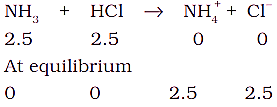The resulting 75 mL of solution contains 2.5 mmol of {tex}\mathrm{NH}_{4}^{+}{/tex} ions (i.e., 0.033 M) and 2.5 mmol (i.e., 0.033 M ) of neutralised NH3 molecules. This NH3 exists in the following equilibrium: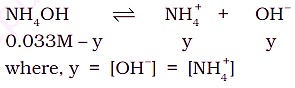The final 75 mL solution after neutralization already contains 2.5 m mol {tex}\mathrm{NH}_{4}^{+}{/tex} ions (i.e. 0.033M), thus total concentration of {tex}\mathrm{NH}_{4}^{+}{/tex} ions is given as:
[{tex}\mathrm{NH}_{4}^{+}{/tex}] = 0.033 + y
As y is small, [NH4OH] {tex} \simeq {/tex} 0.033 M and [{tex}\mathrm{NH}_{4}^{+}{/tex}] {tex} \simeq {/tex} 0.033M.
We know,
{tex}K_{\mathrm{b}}=\left[\mathrm{NH}_{4}^{+}\right][\mathrm{OH}] /\left[\mathrm{NH}_{4} \mathrm{OH}\right]{/tex}
= y(0.033)/(0.033) = 1.77 {tex}\times{/tex} 10–5 M
Thus, y = 1.77 {tex}\times{/tex} 10–5 = [OH] {tex}\left[\mathrm{H}^{+}\right]=10^{-14} / 1.77 \times 10^{-5}=0.56 \times 10^{-9}{/tex}
Hence, pH = 9.24

OR

The given reaction is:
{tex}{N_2}(g) + 3{H_2}(g) \rightleftharpoons 2N{H_2}(g){/tex}
According to available data.
[N2] = [3.0]; [H2 ] = [2.0] and [NH3] = [0.50] {tex}{Q_c} = \frac{{{{[N{H_3}(g)]}^2}}}{{[{N_2}(g)]{{[{H_2}(g)]}^3}}}{/tex}{tex} = \frac{{{{[0.50]}^2}}}{{[3.0]{{[2.0]}^3}}} = \frac{{0.25}}{{24}} = 0.0104{/tex}
The value of the reaction quotient is smaller than the value of the equilibrium constant.
Since the value of Qis less than that of K(0.061), the reaction is not in a state of equilibrium. It will proceed in the forward direction till Qc becomes the same as Kc.

1. There is no neutron present in hydrogen atom. It consists of only one proton.
2. Given, 10-8 mol dm-3 solution of HCl.
Here, the concentration of water cannot be neglected since the solution is very dilute.
So, {tex}\left[ {{H_3}{O^ + }} \right] = {10^{ – 8}} + {10^{ – 7}}M{/tex}
So, pH will be less than 7.
3. Blood red colour
4. In trans-but-2-ene, the dipole moments of the two C—CH3 bonds are equal and opposite and therefore, they cancel out each other.
Hence, trans-2-butene is non-polar.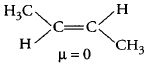5. The shape of s-orbital is spherically symmetrical i.e. it is non–directional in nature.

## Marking Scheme for the Class 11 exam

SubjectBoard MarksPractical or internal Marks
English80 Marks20 Marks
Hindi80 Marks20 Marks
Mathematics80 Marks20 Marks
Chemistry70 Marks30 Marks
Physics70 Marks30 Marks
Biology70 Marks30 Marks
Computer Science70 Marks30 Marks
Informatics Practices70 Marks30 Marks
Chemistry80 Marks20 Marks
Economics80 Marks20 Marks
History80 Marks20 Marks
Political Science80 Marks20 Marks
Geography70 Marks30 Marks
Sociology80 Marks20 Marks
Physical Education70 Marks30 Marks
Home Science70 Marks30 Marks

## CBSE Sample Papers for Class 11

To download sample paper for class 11 Physics, Chemistry, Biology, History, Political Science, Economics, Geography, Computer Science, Home Science, Accountancy, Business Studies and Home Science; do check myCBSEguide app or website. myCBSEguide provides sample papers with solution, test papers for chapter-wise practice, NCERT solutions, NCERT Exemplar solutions, quick revision notes for ready reference, CBSE guess papers and CBSE important question papers. Sample Paper all are made available through the best app for CBSE students and myCBSEguide website.Test Generator

Create question paper PDF and online tests with your own name & logo in minutes.myCBSEguide

Question Bank, Mock Tests, Exam Papers, NCERT Solutions, Sample Papers, Notes